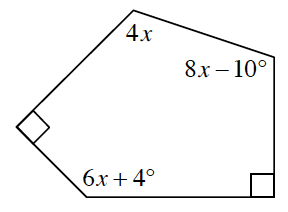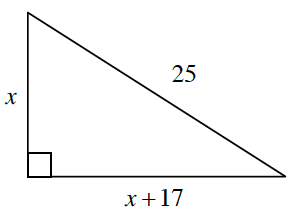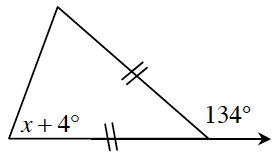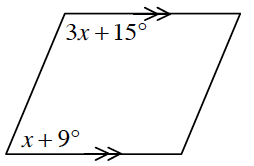### Home > GC > Chapter 11 > Lesson 11.1.3 > Problem11-45

11-45.

​ Write and solve an equation from the geometric relationships provided in the diagrams below.

1.Sum of interior angles $= 180(n − 2) = 180(5 − 2) = 540º$

Therefore, $90 + 4x + (8x − 10) + 90 + (6x + 4) = 540º$

1.$x^2 + (x + 17)^2 = 25^2$

$x^2 + (x^2 + 34x + 289) = 625$

You can ultimately solve this problem by factoring.

$x = 7$

1.Note that because the figure is an isosceles triangle, two angles are equal to $x + 4º$ and the remaining angle is equal to $46º$, since it is supplementary to the angle of $134º$.

$x = 63º$

1.$(3x + 15) + (x + 9) = 180º$